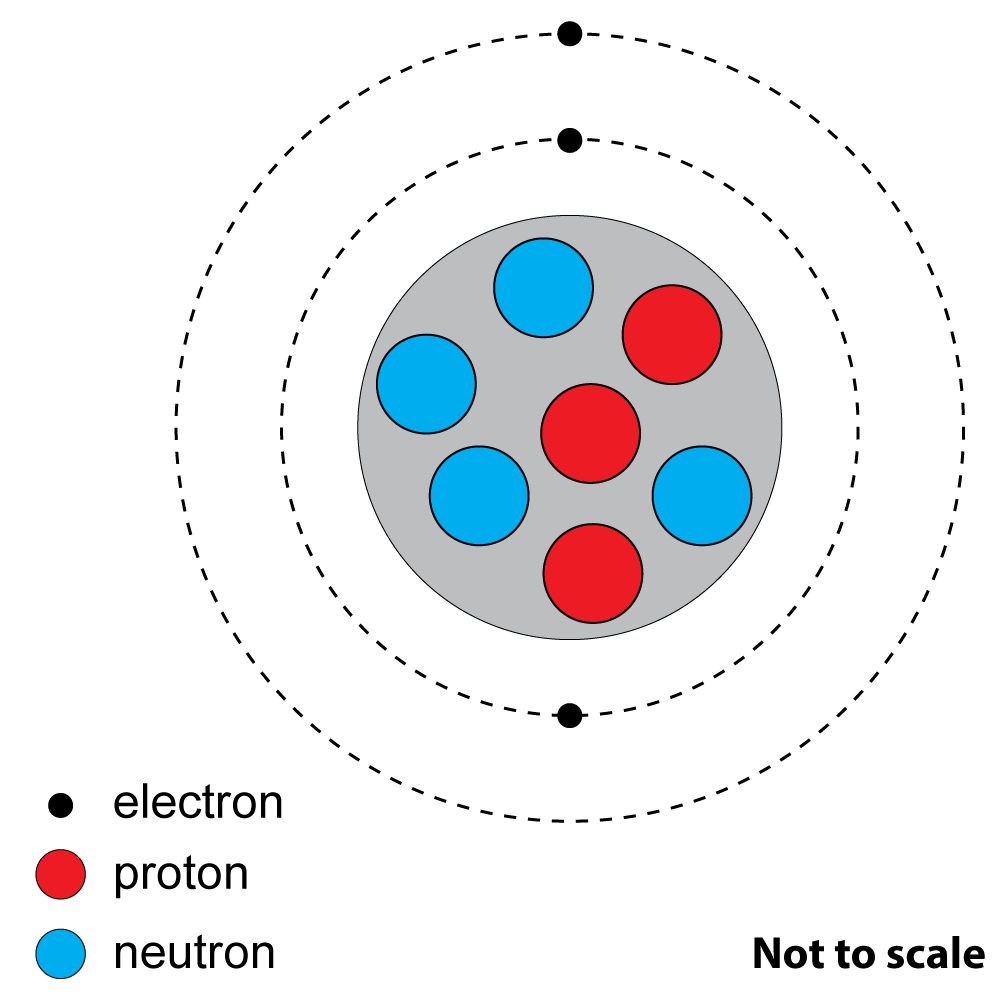Page 1

 NEED HELP? Edexcel iGCSE digital textbooks From the makers of GradeGorilla. GGebooks.com (Opens in a new tab)1. An atom of 14 C contains protons and neutrons. 6

How many?

 number of neutrons number of protons A. 14 6 B. 8 6 C. 6 8 D. 6 14

2+3. Look at this diagram of an atom of a common element.2. What is the atomic (proton) number?

A). 3
B). 4
C). 7
D). 10

3. What is the mass (nucleon) number?

A). 3
B). 4
C). 7
D). 10

4. Two isotopes of the same element have

A). the same number of protons but different numbers of neutrons.
B). the same number of protons but different numbers of electrons.
C). the same number of neutrons but different numbers of protons.
D). the same number of electrons but different numbers of protons.

5. A radioactive isotope is a substance that

A). will eventually gain electrons through bonding.
B). will become stable by cooling down.
C). is unstable and will ‘decay’ by losing particles.

6. Which of the following best describes the words 'contamination' and 'irradiation'?

 Contamination irradiation A. The unwanted presence of radioactive isotopes The exposure of an object to electromagnetic radiation B. The unwanted presence of radioactive isotopes The exposure of an object to nuclear radiation C. The exposure of an object to electromagnetic radiation The unwanted presence of radioactive isotopes D. The exposure of an object to nuclear radiation The unwanted presence of radioactive isotopes

Which type(s) of radiation match the following descriptions?7. The most ionising.
8. A fast moving electron.
9. Can pass through paper.

10. When Uranium decays it emits an alpha particle, forming an isotope of Thorium. Which of the following decay equations is correct?

 A. 238 U232 Th + 2 α 92 90 2 B. 238 U236 Th + 2 α 92 90 2 C. 238 U234 Th + 4 α 92 90 2 D. 238 U232 Th + 4 α 92 90 2
11. What change takes place to an atom during beta decay?

A). A proton changes into a neutron.
B). A proton changes into an electron.
C). An electron changes into a neutron.
D). A neutron changes into a proton.

12. In this decay equation, what are the missing numbers x and y?

 24 Na24 Mg + y β 11 x -1
• A. x=10, y=1
• B. x=10, y=0
• C. x=12, y=1
• D. x=12, y=0